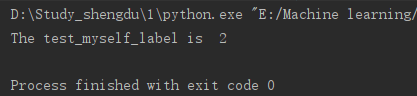# 机器学习实战之k-近邻算法识别手写数字（含拍照检验步骤详解）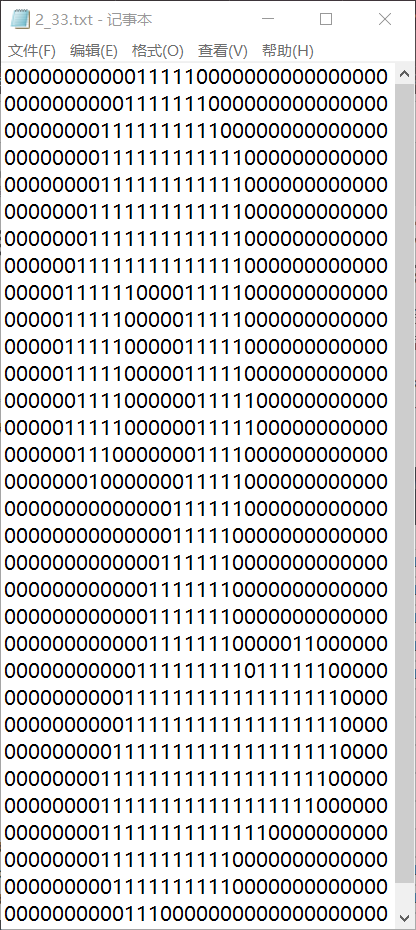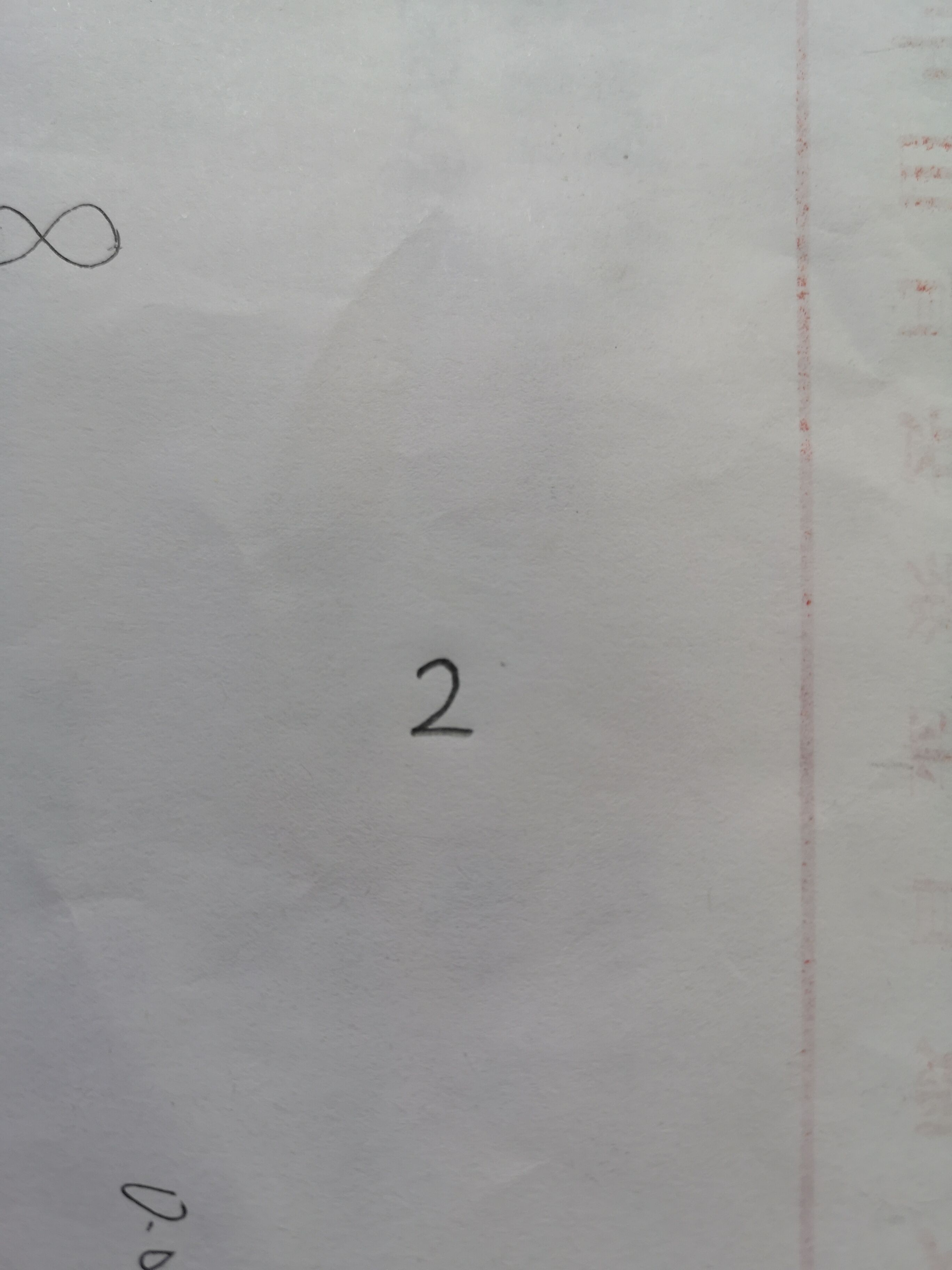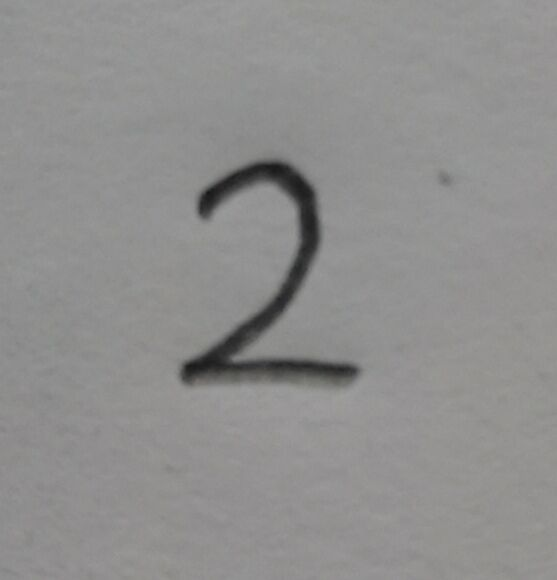1.在ps中打开该图像
2.点击上方的‘图像’——>‘图像大小’——>修改图像大小栏中的‘宽度（W）’与‘高度（H）’，再点击确定

3.点击‘文件’——>‘储存为’，把当前图像储存好。如下图：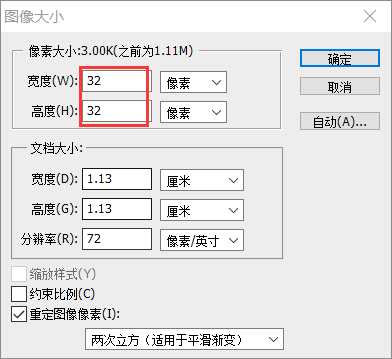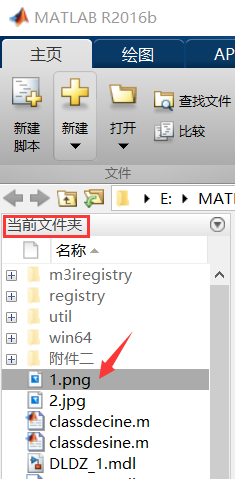MATLAB代码如下：

``````i=imread('1.png');
i1=rgb2gray(i);     %i1灰度图像
i2=im2bw(i1);       % i2是二值图，不需要求阈值。但是i2中黑色部分为0，白色部分为1，与 ’2_33.txt‘ 中刚好相反，因此需要倒转
for j=1:32          %把二值化后的矩阵中0变成1，1变成0
for k=1:32
if i2(j,k)==0
i2(j,k)=1
else
i2(j,k)=0
end
end
end
fid=fopen('test_myself.txt','wt');      %写的方式打开文件（若不存在，建立文件）；
fprintf(fid,'%d',i2');                  %d 表示以整数形式写入数据，注意：此时i2是转置的
fclose(fid);                            %关闭文件
``````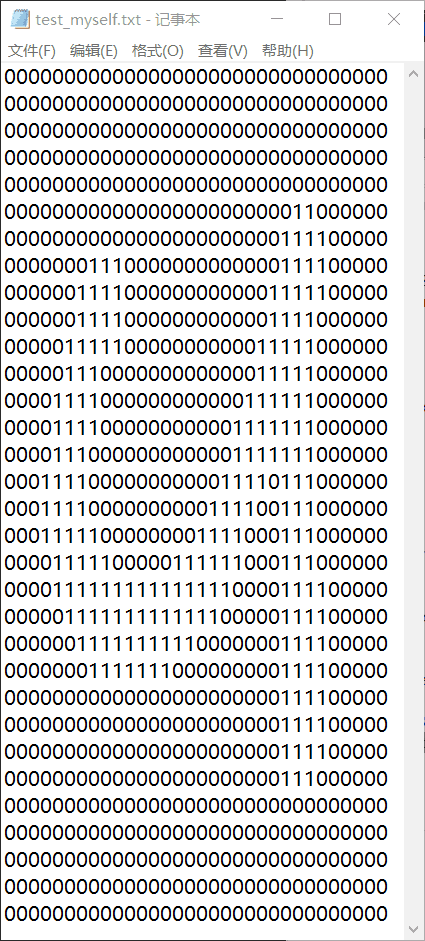``````from numpy import *
import operator
from os import listdir

def classify0(inX, dataSet, labels, k):   #对于该函数的解释，见后面图中
dataSetSize = dataSet.shape
diffMat = tile(inX, (dataSetSize,1)) - dataSet
sqDiffMat = diffMat**2
sqDistances = sqDiffMat.sum(axis=1)  #every hang add together
distances = sqDistances**0.5
sortedDistIndicies = distances.argsort()
classCount={}
for i in range(k):
voteIlabel = labels[sortedDistIndicies[i]]
classCount[voteIlabel] = classCount.get(voteIlabel,0) + 1
sortedClassCount = sorted(classCount.items(), key=operator.itemgetter(1), reverse=True)
return sortedClassCount

def img2vector(filename):          #该函数是针对文本中为32行*32列进行的处理函数，返回一个1*1024的数组
returnVect = zeros((1,1024))
fr = open(filename)
for i in range(32):
for j in range(32):
returnVect[0,32*i+j] = int(lineStr[j])
return returnVect

##
def img_myself(filename):         #该函数是针对文本中为1*1024进行的处理函数，返回一个1*1024的数组
returnVect=zeros((1,1024))
fr=open(filename)
for i in range(1024):
returnVect[0,i]=int(lineStr[i])
return returnVect

def handwritingPerson(filename):  #filename为待测试的txt文件，本例中为'test_myself.txt'文件
hwLabels = []
trainingFileList = listdir('trainingDigits')  #储存训练集'trainingDigits'文件夹中所有的文件名到列表trainingFileList中
m = len(trainingFileList)
trainingMat = zeros((m, 1024))                #每行储存一个图像
for i in range(m):
fileNameStr = trainingFileList[i]         #第i个文件的文件名储存在fileNameStr中，如‘0_0.txt’
fileStr = fileNameStr.split('.')       #把文件名用‘.’分开成两部分，取第一部分，如把‘0_0.txt’分开成‘0_0’与‘txt’两部分，0代表取前一部分
classNumStr = int(fileStr.split('_'))  #同理，取‘0_0’中前一部分0，并转换为int类型
hwLabels.append(classNumStr)              #训练集的标签矩阵
trainingMat[i, :] = img2vector('trainingDigits/%s' % fileNameStr)   #打开trainingDigits文件夹下的文件，读取并转化为1*1024的矩阵后，添加到trainingMat的对应行中，组成训练集的矩阵
test_myself=img_myself(filename)              #读取filename文件并转化为1*1024的矩阵，组成测试集
test_myself_label=classify0(test_myself,trainingMat,hwLabels,5)   #进行训练并测试，把结果放到test_myself_label中
print("The test_myself_label is ",test_myself_label)

``````

classify0()函数的解释如下图：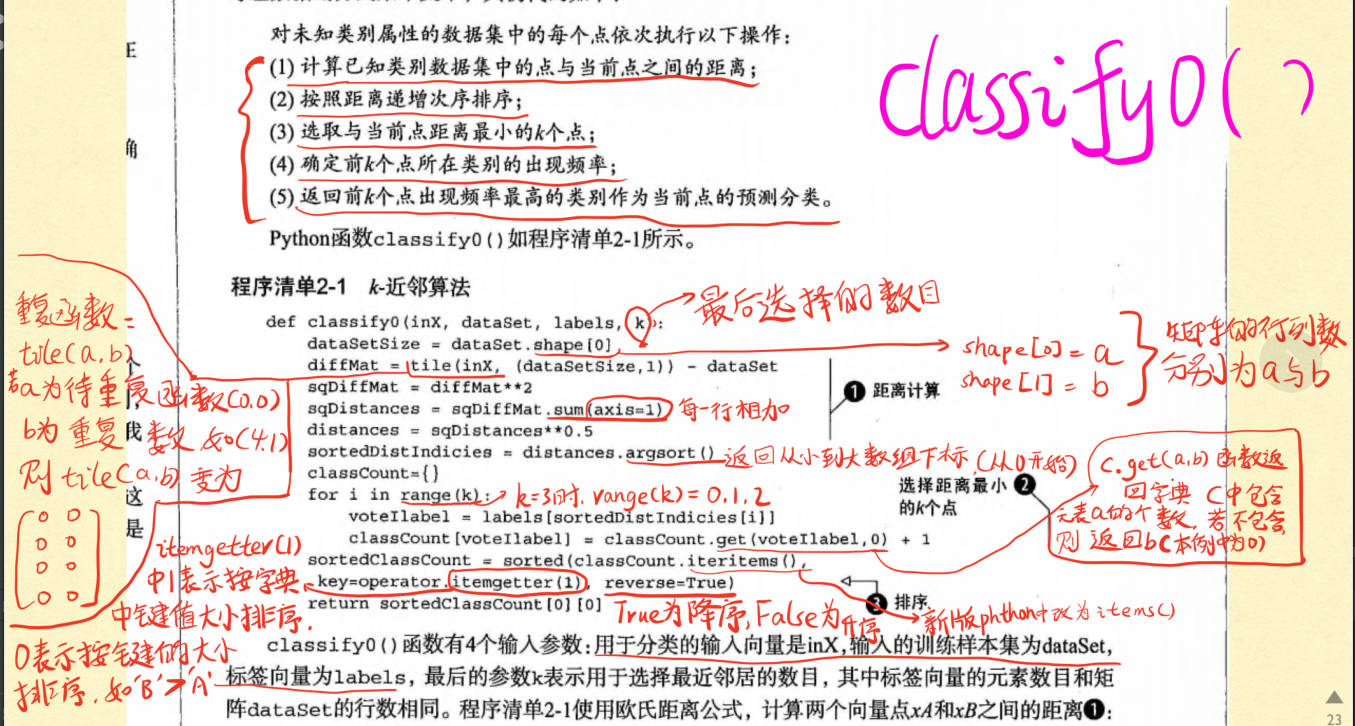``````handwritingPerson('test_myself.txt')
``````